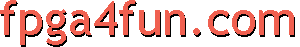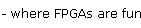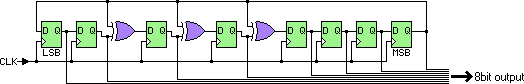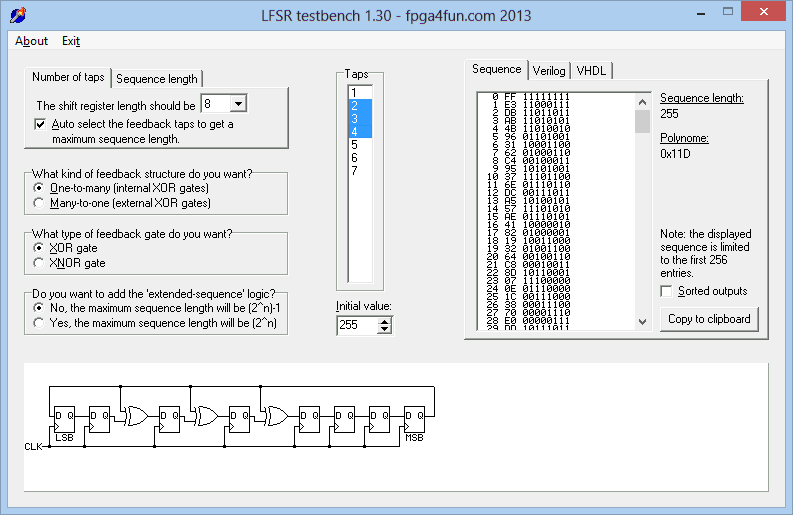Counters 3 - LFSR counters

Let's say you want a counter that counts more or less "randomly", you can use an LFSR.
Here's an example.As you can see, an LFSR is a shift-register with some XOR gates. The one shown above is an 8-taps LFSR (it uses 8 flip-flops).

The output sequence starts as follow (assuming all the flip-flops start at '1'):

11111111
11000111
11011011
11010101
11010010
01101001
10001100
01000110
00100011
10101001
11101100
01110110
00111011
10100101
...

Here's the LFSR source code.

module LFSR8_11D(
input clk,
output reg [7:0] LFSR = 255   // put here the initial value
);

wire feedback = LFSR;

always @(posedge clk)
begin
LFSR <= feedback;
LFSR <= LFSR;
LFSR <= LFSR ^ feedback;
LFSR <= LFSR ^ feedback;
LFSR <= LFSR ^ feedback;
LFSR <= LFSR;
LFSR <= LFSR;
LFSR <= LFSR;
end
endmodule

Notice that we made it start at 255. That's because 0 is a dead-end state, so we can choose any start value but 0. After that, the LFSR changes value at each clock cycle but never reaches 0, so it goes through only 255 8bit values (out of 256 possible combinations of a 8bit output) before starting again.

Now, instead of outputting the full eight bits, we could output just one bit (i.e. choose any of the flip-flops to make the output, keep the other seven internal). That generates a string of 255 0's and 1's that looks random (although it repeats itself after 255 clocks). Useful for a noise generator...

Customization

You can tweak the LFSR:

• Select a different number of taps (we chose 8 above).
• Change the way the feedback network is wired - like change the number XOR gates, where they are placed, or replace XOR gates by XNOR.

Certain feedback configurations will create islands of possible values. For example, this LFSR looks similar to the first one but loops through only 30 values.

module LFSR8_105(
input clk,
output reg [7:0] LFSR = 255
);

wire feedback = LFSR;

always @(posedge clk)
begin
LFSR <= feedback;
LFSR <= LFSR;
LFSR <= LFSR ^ feedback;
LFSR <= LFSR;
LFSR <= LFSR;
LFSR <= LFSR;
LFSR <= LFSR;
LFSR <= LFSR;
end
endmodule

It is also possible to add a bit of logic in the feedback so that the LFSR reaches all possible states.

module LFSR8_11D(
input clk,
output reg [7:0] LFSR = 255
);

wire feedback = LFSR ^ (LFSR[6:0]==7'b0000000);  // modified feedback allows reaching 256 states instead of 255

always @(posedge clk)
begin
LFSR <= feedback;
LFSR <= LFSR;
LFSR <= LFSR ^ feedback;
LFSR <= LFSR ^ feedback;
LFSR <= LFSR ^ feedback;
LFSR <= LFSR;
LFSR <= LFSR;
LFSR <= LFSR;
end
endmodule
LFSR testbench

We made a small Windows utility that allows experimenting with LFSR designs.